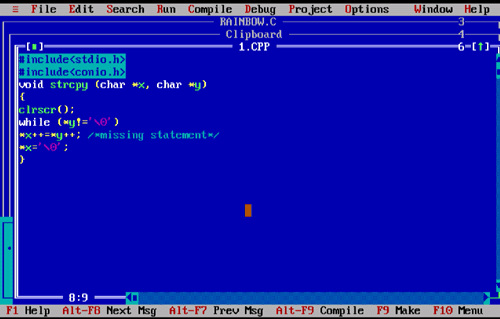# C Pointer MCQs - Multiple Choice Questions with Answers

Questions
1 Given the following code fragment:

```	main()
{
int row,i,sum=0;
int *p=row;
for(i=0;i<20;i++)
*(p+i)=1;
for(i=0;i<20;i+=sizeof(int))
sum+=*(p+i);
printf("sum=%d\n",sum);
}
```

What will be the result of execution?

A sum=10
B sum=40
C sum=60
D sum=190

2 What is the missing statement in the following function which copies string x into string y?

```	void strcpy (char *x, char *y)
{
while (*y!='\0')
................./*missing statement*/
*x='\0';
}
```

What will be the result of execution?

A x=y
B *x++=*y++
C (*x)++=(*y)++
D none of these

Pointer variable char *x is pointing to a location and the char *y is assigned to that location. If we assume the missing statement is *x++=*y++ then both the variables point to the next respective location till null ('\0') found.3

```	char *ptr;
char myString[]="abcdefg";
ptr=myString
ptr+=5;
```

The pointer ptr points to which string?

A fg
B efg
C defg
D cdefg

ptr+=5 means ptr=ptr+5

That means the pointer variable is incremented by 5. Hence it is pointing to the 6th location. i.e. fg

4 Output of the following program will be

```	main()
{
int a[]={1,2,9,8,6,3,5,7,8,9};
int *p=a+1;
int *q=a+6;
printf("\n%d",q-p);
}
```
A 9
B 5
C 2
D None of these5 Given float *pf; int *pi; Which of the following is true?
A sizeof(pf) > sizeof(pi)
B sizeof(pi) < sizeof(pf)
C sizeof(pf) == sizeof(pi)
D None of these above